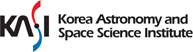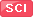Journal Paper

전체
Self-consistent potential-density pairs of thick discs and flattened galaxies• Jin Hyeok AN; N. W. Evans;
• 2019-07-01
• MONTHLY NOTICES OF THE ROYAL ASTRONOMICAL SOCIETY 486 3 : 3915~3926
We analyse the Miyamoto？Nagai substitution, which was introduced over 40 years ago to build models of thick discs and flattened elliptical galaxies. Through it, any spherical potential can be converted to an axisymmetric potential via the replacement of spherical polar r^2 with R^2+(a+√(z^2+b^2))^2 where (R, z) are cylindrical coordinates and a and b are constants. We show that if the spherical potential has everywhere positive density, and satisfies some straightforward constraints, then the transformed model also corresponds to positive density everywhere. This is in sharp contradistinction to substitutions like r^2 → R^2 + z^2/q^2, which leads to simple potentials but can give negative densities. We use the Miyamoto？Nagai substitution to generate a number of new flattened models with analytic potential-density pairs. These include (i) a flattened model with an asymptotically flat rotation curve, which (unlike Binney’s logarithmic model) is always non-negative for a wide-range of axis ratios, (ii) flattened generalizations of the hypervirial models which include Satoh’s disc as a limiting case and (iii) a flattened analogue of the Navarro？Frenk？White halo which has the cosmologically interesting density fall-off of (distance)^(？3). Finally, we discuss properties of the prolate and triaxial generalizations of the Miyamoto-Nagai substitution.